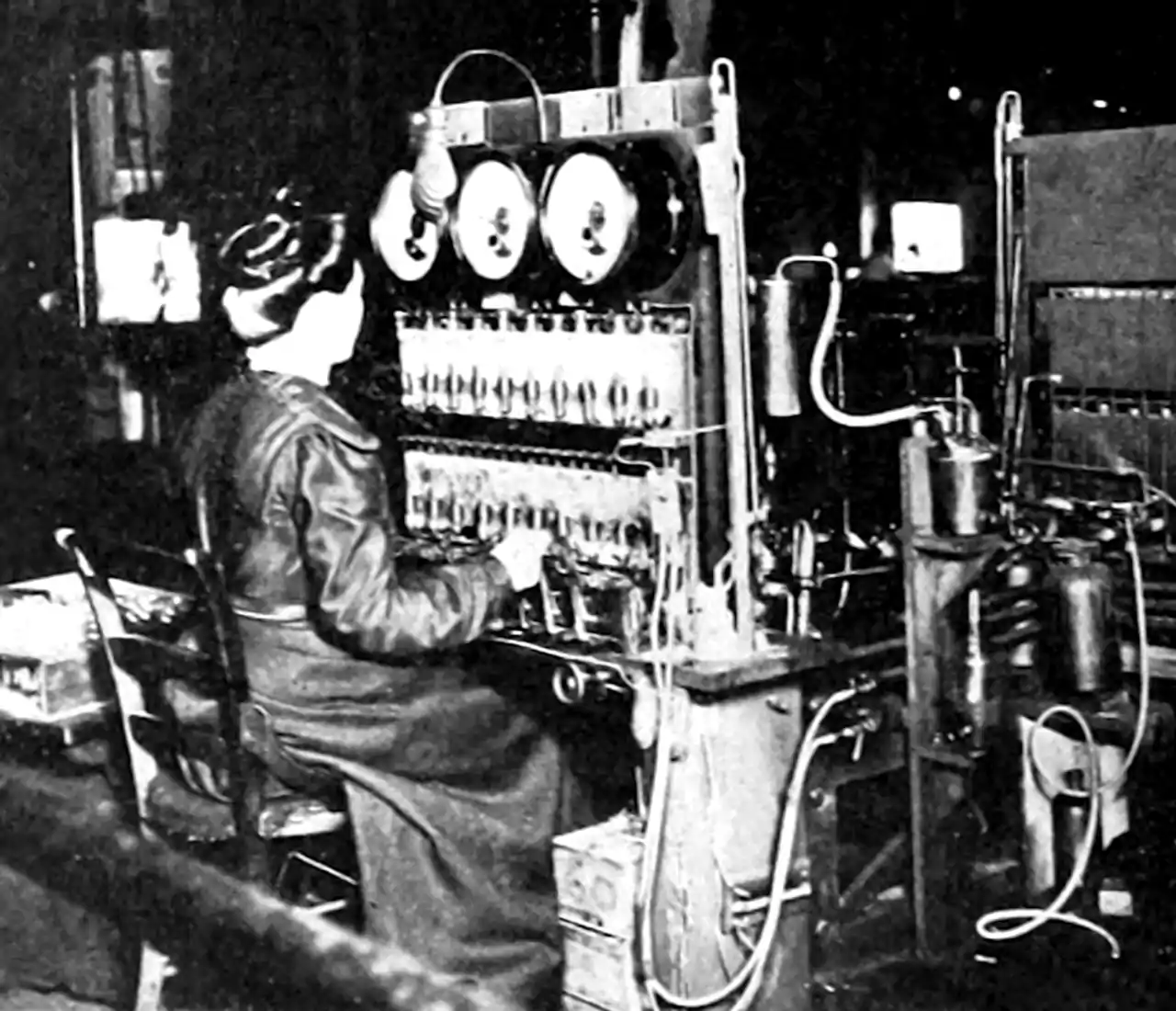# Vector Gaussian processsesAs scalar Gaussian processes are to GP regression so are vector Gaussian processes to vector GP regression.

We recall that a classic Gaussian random process/field over some index set $$\mathcal{T}$$ is a random function $$f:\mathcal{T}\times\mathcal{T}\to\mathbb{R},$$ specified by the (deterministic) functions giving its mean $m(t)=\mathbb{E}\{f(t)\}$ and covariance $K(s, t)=\mathbb{E}\{(f(s)-m(s))(f(t)-m(t))\}.$

We can extend this to multivariate Gaussian fields $$\mathcal{T}\times\mathcal{T}\to\mathbb{R}^d$$. This exposition follows .

For such fields we require that $$\left\langle\alpha, f_{t}\right\rangle$$ is a real valued Gaussian field for every $$\alpha \in \mathbb{R}^{d}$$. In this case, the mean function is now $$m:\mathcal{T}\to\mathbb{R}^{d}$$ and the covariance function now $$K:\mathcal{T}\times\mathcal{T}\to \mathbb{R}^{d \times d}$$ matrices where every $$K(s,t) must be positive definite.$$ In statistical practice we refer to a matrix $$K(s,t)$$ by various names, but in my office we talk about cross-covariance. It has the interpretation $K(s, t)=\mathbb{E}\left\{(f(s)-m(s))^{\top}(f(t)-m(t))\right\}.$ The individual elements of $$K$$ are thus given by $K_{i j}(s, t)=\mathbb{E}\left\{\left(f_{i}(s)-m_{i}(s)\right)\left(f_{j}(t)-m_{j}(t)\right)\right\} .$ We now have consider two positive-definitenesses:

1. For each fixed pair $$s, t$$, the matrix $$K(s, t)$$ is positive-definite.
2. for each pair $$i, j$$, the function $$K_{i j}:\mathcal{T} \times \mathcal{T}\to\mathbb{R}$$ is positive-definite.

We know that finding positive-definite scalar-valued functions can be difficult. So finding positive-definite matrix-valued functions feels like it might also be a burden both to our monkey brains and/or even our computation power.

We can come up with other perspectives on this construction. One version of this is given by Eric Perim, Wessel Bruinsma, and Will Tebbutt, in Gaussian Processes: from one to many outputs.

In their version, if we want to model $$p$$ outputs over some input space $$\mathcal{T}$$ we construct an extended input space: $$\mathcal{T}_{\text {ext }}=\{1, \ldots, p\} \times \mathcal{T}$$. Then, the vector GP’s mean function and covariance functions take inputs in the extended space $$m: \mathcal{T}_{\text {ext }} \rightarrow \mathbb{R}$$, and $$K: \mathcal{T}_{\text {ext }}^{2} \rightarrow \mathbb{R}.$$ i.e. we still have a GP, but with inputs handled in a clever way. This does not, to me, make it easier to define covariance kernels, but it makes them look different.

Both of these constructions are useful in using multi-output kernels in practice. Have a look at some details from a computational perspective over at vector GP regression.

### No comments yet. Why not leave one?

GitHub-flavored Markdown & a sane subset of HTML is supported.